Analysis Control Created Edited

# Construction Stage Analysis Control

## Function

• Here are the analysis conditions required to perform analysis for each construction stage and fulfill the analysis functionalities.
• A civil structure such as a suspension bridge, cable stayed bridge or PSC (prestressed or post-tensioned concrete) bridge requires separate and yet inter-related analyses for the completed structure and interim structures during the construction. Each temporary structure at a particular stage of construction affects the subsequent stages. Also, it is not uncommon to install and dismantle temporary supports and cables during construction. The structure constantly changes or evolves as the construction progresses with varying material properties such as modulus of elasticity and compressive strength due to different maturities among contiguous members. The structural behaviors such as deflections and stress re-distribution continue to change during and after the construction due to varying time dependent properties such as concrete creep, shrinkage, modulus of elasticity (aging) and tendon relaxation. Since the structural configuration continuously changes with different loading and support conditions, and each construction stage affects the subsequent stages, the design of certain structural components may be governed during the construction. Accordingly, the time dependent construction stage analysis is required to examine each stage of the construction, and without such analysis the analysis for the final stage alone will not be reliable.
• midas Civil considers the following items for a Construction Stage analysis

• Time dependent material properties

1. Creep effects of concrete members having different maturities

2. Shrinkage effects of concrete members having different maturities

3. Time dependent compressive strength gain for concrete members

4. Prestressing tendon relaxation

• Expression of Construction Stages

1. Creation (activation) and deletion (deactivation) of members with certain maturities.

3. Change of boundary conditions relative to time

• midas Civil follows the procedure noted below for a construction stage analysis reflecting time dependent properties.

1. Open the dialog box for "Analysis > Construction Stage Analysis Control" and specify the types of time-dependent material properties to consider for the construction stage analysis. Also, specify the number of iterations and convergence criteria required for creep calculations.

2. If the analysis model represents a PSC (prestressed or post-tensioned concrete) structure, specify whether to consider the effects of tendon relaxation.

3. Click on "Analysis > Perform Analysis" to execute the analysis.

4. Once the analysis is successfully completed, navigate to the "Result" menu to review the stress, member forces, and displacements for each construction stage.

## Call

From main menu, select [Analysis] tab > [Analysis Control] group >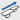[Construction Stage]

## Input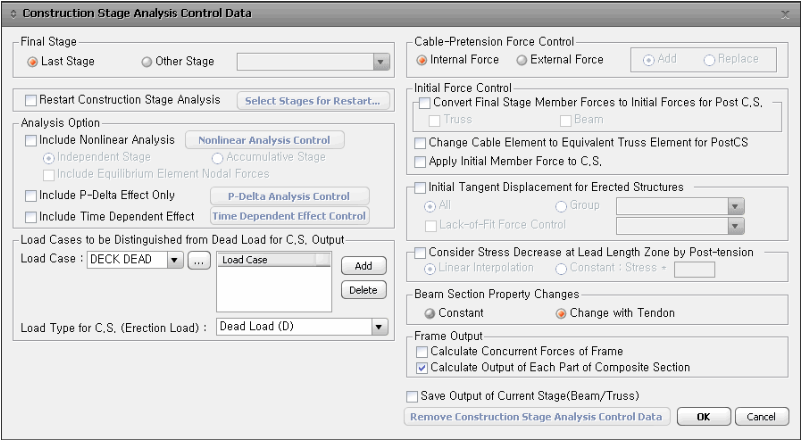Construction Stage Analysis Control dialog box

### Final Stage

To perform analysis for a specific construction stage or the final stage of the structure, you can select the desired construction stage to be considered. Typically, the analysis is carried out until the final construction stage. However, it is also possible to limit the analysis to a specific construction stage specified by the user.

Last Stage

Assign the last stage as the true last stage.

Other Stage

Assign a stage within the overall construction stages as the final stage.

### Restart Construction Stage Analysis

Restart the construction stage analysis from the stage specified by the user. Restarting the analysis from the modified stage will save time.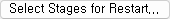: Select the stages to be used for Restart.

• In order to use Restart function, analysis results corresponding to each construction stage should be stored in DB. It would be effective, in terms of time and storage, to select only the stages that are necessary for Restart and to save intermediate files just in case the program stops.
• Restart function is performed using the results obtained from the previously performed analysis. When there are some changes that can affect the results of the stages after which Restart function is applied, the analysis results may not be consistent.

When some changes are made to the structural system of the stages after which Restart function is to be applied

When some changes are made to the boundary conditions and loadings of the stages after which Restart function is to be applied

When some changes are made to the material or sectional properties that are used throughout the entire stages

• The consistency of the analysis results using Restart function is dependant upon the input data. Therefore, as far as the final result is concerned, it is recommended to perform analysis from the start to end stages rather than using the Restart function.

### Analysis Option

Include Nonlinear Analysis

Perform construction stage analysis, including geometrically nonlinear analysis to account for changes in the geometry.

Click on the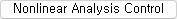after checking the "Nonlinear Analysis control" and enter the following information in the dialog box below:a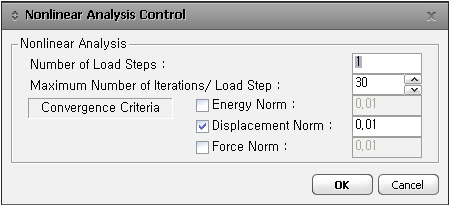Maximum number of iterations of analysis per Load Step.

Convergence Criteria

Specify the basis on which to assess the convergence. Enter the norm values for Energy (Member force x displacement), displacement and member forces.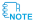The selection of the convergence criteria for repeating or ending the analysis must be based on the condition to reflect the effects of various degrees of freedom. For example, in the case of Displacement Norm, if the displacement resulting from the corresponding analysis step is {D1}, and the total displacement accumulated from each step is {D2}, the Norm is expressed as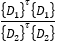.

If this value is smaller than the specified value, a convergence is considered to have occurred and the program stops the iterative analysis.

Independent Stage

In each construction stage, we construct an independent model and perform the analysis. It is not possible to simultaneously consider geometric nonlinearity and time-dependent effects in the independent model analysis. Other options, excluding the nonlinear analysis control options (part of the dialog box), cannot be set. This option is used for the reverse construction stage analysis of a suspension bridge considering large deformations.

Accumulative Stage

We perform nonlinear analysis while accumulating the results of each construction stage. In the accumulative model geometrically nonlinear analysis, we can consider time-dependent effects, select the Pretension Type of cables, and calculate tangent displacements (including Lack of Fit Force). This option is mainly used for forward construction stage analysis considering large deformations in cable-stayed bridges.

Include Equilibrium Member Force

We consider equilibrium forces to perform nonlinear analysis. It is used in the reverse construction stage analysis of a suspension bridge, considering initial equilibrium forces based on the forces generated in the completed state.Geometric Nonlinear Accumulated Stage Analysis

1. Geometric nonlinear analysis should be performed based on Real Displacement. Therefore, "Initial Tangent Displacement for Erected Structures" option should be checked on. Even if the user does not check this option and clicks OK, the program internally will apply this option.

2. For the geometric nonlinear analysis in cable stayed bridge, elements except for cable elements cannot be installed, loads other than cable pretension cannot be active and boundary conditions cannot be active in the stage at which cable elements are installed.

3. In the case when supports (links, springs, etc.) become activated during construction stage, the supports should be activated earlier than the elements connected to the elements. If the elements are activated earlier than the supports, the supports should be activated with the "Original" option selected at the next stage. If the elements and supports are activated at the same time, displacements occurring at the elements are transferred to the boundary conditions and accordingly, incorrect results may be obtained.Analysis result is produced at both I and J ends only in geometry nonlinear analysis.

Include P-Delta Effect Only

Consider geometric stiffness due to axial forces. It cannot be performed simultaneously with nonlinear analysis.

Click on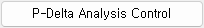after checking on "Include P-Delta Effect Only" under the Analysis Option and enter the following data in the dialog boxNumber of Iterations : Maximum number of iterations of analysis

Convergence Tolerance : Tolerance for convergence

Include Time Dependent Effect

If "Include Time Dependent Effect" is checked on in the Analysis Option, Click the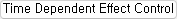to get the dialog box to input the time dependent information.

Define the material properties related to creep and shrinkage in Time Dependent Material(Creep/Shrinkage)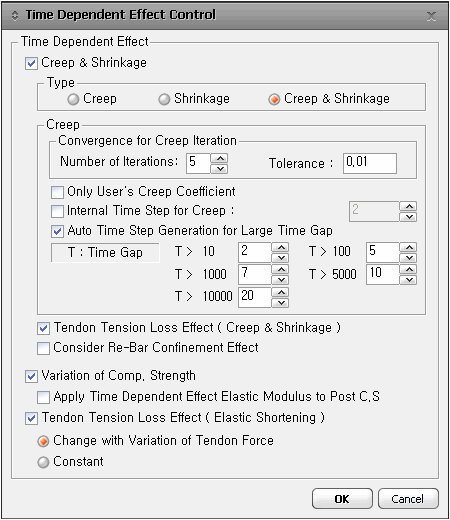Creep & Shrinkage

When considering creep and dry shrinkage, check this and input followings :

Type

Select one of the options for considering creep and/or shrinkage.

Creep

Convergence for Creep Iteration

Specify the convergence requirement for ending the repetitive process in the analysis reflecting creep.

Number of Iterations : Maximum number of repetitions

Tolerance : for convergence

Only User's Creep Coefficient

Perform the construction stage analysis only using the creep coefficients entered by the user. Creep coefficients are entered by elements in Creep Coefficient for Construction Stage.

Internal Time Step for Creep

Specify a number, which is used to divide a construction stage to create internal steps for considering creep.Internal Steps are applied in the process of analysis, and the corresponding analysis results are not produced.

Auto Time Step Generation for Large Time Gap

Specify a number, which is used to divide a construction stage to create internal steps when the duration of the construction stage is too long.Internal Steps are applied in the process of analysis, and the corresponding analysis results are not produced.

Tendon Tension Loss Effect(Creep & Shrinkage)

Check on to reflect the effect of prestressing tension loss of tendons due to creep and shrinkage. Define the tendon properties of the prestressing loss in "Tendon Property".

Consider Reinforcement Confinement Effect

Check on to reflect the effect of rebar confinement for creep and shrinkage. Rebar data can be defined in "Section Manager".

Variation of Comp. Strength

Check on to reflect the change of modulus of elasticity related to the change of compressive strength of concrete. The maturity variant property is defined in "Time Dependent Material(Comp. Strength)".

Apply Time Dependent Effect Elastic Modulus to Post C.S

If checked, the final stage's elastic modulus will be applied in the Post Construction Stage.

If unchecked, the elastic modulus from the DB Material will be applied in the Post Construction Stage.

Tendon Tension Loss Effect(Elastic Shortening)

Check on to reflect the prestressing tension loss of tendons due to the elastic deformations of concrete.

Dead Load is generally the most significant component of all the loads applied to construction stage analysis. The results of all the load cases except for Creep, Shrinkage and Relaxation of Tendons are lumped into CS: Dead Load. Here we can select certain load cases to be distinguished from the Dead Load and produce the results under CS: Erection Load.

Load Type for CS(Erection Load) : Specify a load type classified into CS:Erection, which is differently categorized from CS:Dead. This is helpful in distinguishing the pre-composite dead load from the post-composite long term loads for the Steel Composite design as per AASHTO code. This is effective when the Auto Generation function is used for generating the load combinations.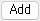: Add an erection load case to be distinguished from Dead Load for C.S. Output.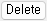: Delete the selected erection load cases from the list.When performing construction stage analysis, the following Load Cases are automatically generated as results: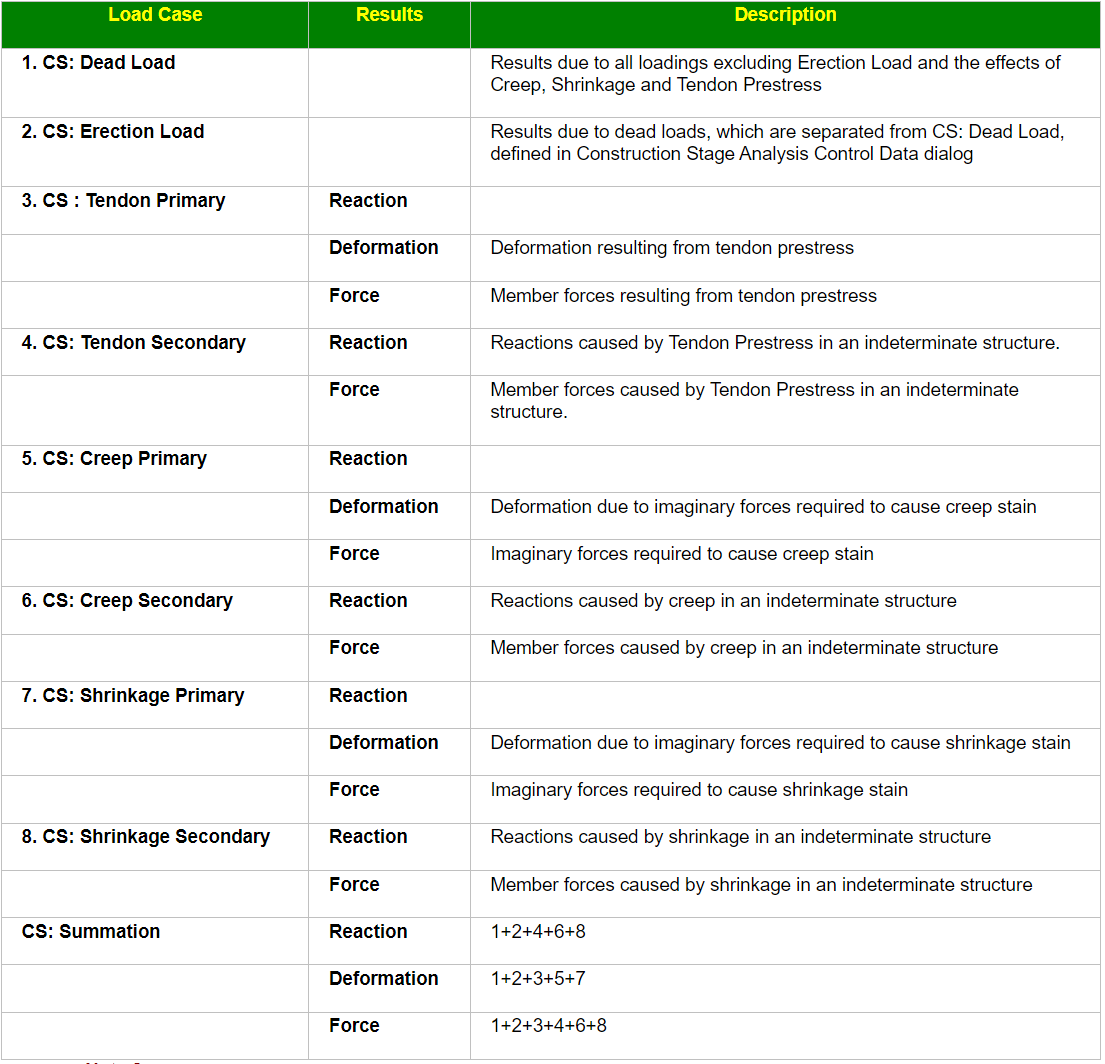Tendon Primary(CS)와 Secondary(CS)
Tendon Primary represents member forces caused by Tendon Prestress forces. Tendon Secondary represents member forces resulting from Tendon Prestress forces acting in an indeterminate structure. To check analysis results, Primary and Secondary can be regarded as internal forces and external forces respectively. For design, however, the program internally recalculates member forces due to Primary considering the translation of neutral axis so as to use them as internal forces, and member forces due to Secondary are used as external forces.

### Cable-Pretension Force Control

Define the method of applying the pretension forces in cable elements.

Internal force : Apply the pretension forces as internal forces (which change after redistribution within the structure in the current construction stage).

External force : Apply the pretension forces as external forces forces (which remain unchanged in the current construction stage).Pretension load is based on the concept of imposing deformation on the truss element in a structure. The method of introducing such deformation is tantamount to installing a truss element shorter than the length of the original element. The length is shorter by the magnitude of deformation. Once a shorter length element is installed in the overall structure, it has an effect of pulling the structure from the nodes to which the element is attached. The deformation is determined by the force and the stiffness of the truss element. (L = (P*L)/(E*A))

Pretension load of Internal Type is applied as deformation in the member equivalent to the pretension load, and the overall structure is analyzed. The resulting member force depends on the stiffness of the end nodes to which the member is attached. Upon applying the deformation to the truss member, large nodal displacements of the end nodes would imply a small member force, and vice versa. If the end nodes are fixed, the resulting member force will be equal to the specified pretension load. When other loads are acting on the member in the same load case, the principle of superposition applies.

Pretension load of External Type is applied to a member when we wish to impose the member to be subjected to the specified pretension load, while the overall structure is analyzed. This leads to the applied pretension load becoming equal to the resulting member force. When a pretension load is inducted into a cable in a particular construction stage, we are controlling the tension in the cable as opposed to controlling its deformation to a uniform magnitude. The External Type load has been implemented so that a specific tension force can be maintained in a particular cable in a particular construction stage. If other loads are applied to the member in the same load case, the resulting member force will remain constant as the pretension load.

The External Type load can be used only in the current construction stage. The options, Add and Replace, are provided to handle when pretension load is input more than once. If Add is checked on, any subsequent pretension load applied to a member will be added to the pre-existing force in the member. If Replace is selected, a pretension load applied to a member is maintained considering the pre-existing member force. The program automatically computes the additional tension force to make the tension force in the member equal to the specified pretension value.

Since a pretension is a loading, it is independent of the stiffness of the structure. Even in the case of the cable element, whose stiffness changes with tension forces, pretension is treated as a regular load.

Add: Add external pretension forces to the pre-existing tension forces of cable elements.

Replace: Replace the pre-existing tension forces of cable elements with applied external pretension forces.If the initial pretension forces are applied as internal forces, the forces in the cable elements become reduced due to the deformation of the support structure based on its stiffness. If the initial pretension forces are applied as external forces, the forces are treated as external loads to the support structure at the construction stage of pretensioning; hence the forces in the cable elements remain unchanged as the initial pretension forces at the corresponding construction stage.

When applying external forces, there are two methods: "Add" and "Replace."
In the "Add" method, when applying external forces, the forces are combined with the existing member forces (tensions). This combined force determines the cable tension, taking into account the existing member forces.
In the "Replace" method, the external forces are applied independently and replace the existing member forces. The cable tension is determined solely based on the externally applied forces, without considering the existing member forces.

### Initial Force Control

Convert Final Stage Member Forces to Initial Forces for Post C.S.

To incorporate the geometric stiffness due to cable forces from the last step of the construction stage analysis into the subsequent post-construction stage or completed stage analysis, the member forces are converted to initial forces. The converted member forces from the last step are output in the "Load > Initial Forces > Small Displacement > Initial Element Forces (CS)" table, and the resulting values are considered in the post-construction stage analysis.

After performing forward analysis for a cable-stayed bridge or a suspension bridge during the construction stage, it is possible to perform the completed stage analysis, considering the geometric stiffness induced by cable forces from the last step.

If both the Initial Element Forces table and Initial Element Forces (CS) table have values inputted simultaneously, the user-inputted values in the Initial Element Forces table take precedence. However, if the "Apply Initial Member Force to C.S." option is checked, the member forces from the last step of the construction stage (Initial Element Forces (CS)) are always applied in the post-construction stage analysis.

Truss : Applicable for Truss and Cable elements (When Include Nonlinear Analysis option is activated)

Beam : Applicable for Beam elements

Change Cable Element to Equivalent Truss Element for PostCS

In the analysis of cable-supported bridges such as suspension bridges and cable-stayed bridges, various types of loads are considered in the post-construction stage (PostCS) or completed stage analysis. These load types include linear static loads (ST), moving loads (MV), point load settlements (SM), response spectrum loads (RS), and others. Some of these load types, like moving loads (MV), follow the principle of linear superposition.

Since load types with changing stiffness cannot be analyzed using cable elements, the cable elements are automatically replaced by truss elements. However, for linear static loads (ST), which include linear superposition, the characteristics of the cable elements are considered. In nonlinear analysis, elastic stiffness is considered, while in linear analysis, equivalent truss analysis is used.

Combining loads with different stiffnesses during design is not a correct approach. Therefore, even for linear static loads (ST), they are analyzed using truss elements instead of cable elements in the PostCS stage, allowing combination with moving loads, point load settlements, and other loads. However, the tension of the cable element from the last step of the construction stage is utilized to calculate the equivalent stiffness of the truss used in PostCS. This allows for a linear analysis that considers the nonlinearity of the cable elements as much as possible.

Check on : Equivalent cable element stiffness is calculated using the tensions in the cable elements at the last construction stage. The equivalent stiffness is then used to analyze all the load cases in Post CS. Change in stiffness due to changes in tensions in the cable elements is not recalculated for linear load combination. By checking this function, the same stiffness can be applied to various load cases at Post CS.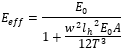Check off : The stiffness calculation method for cable elements varies depending on the type of load. When subjected to linear static loads (ST), the element characteristics are determined based on the analysis type (elastic catenary in nonlinear analysis, equivalent truss in linear analysis). For moving loads (MV), point load settlements (SM), response spectrum loads (RS), and other loads, they are analyzed using linear truss elements without considering the geometric stiffness.

Apply Initial Member Force to C.S.

The Initial Forces entered in the Initial Element Forces are reflected in the construction stage forces. They are applied in the first construction stage where the corresponding element becomes active. When you want to perform construction stage analysis starting from an arbitrary stage, you can reflect the accumulated member forces up to that point as Initial Forces.

When both the "Apply Initial Member Force to C.S." option and the "Convert Final Stage Member Forces to Initial Force for Post C.S." option are selected, during the construction stage, the Initial Forces entered in the Initial Element Forces table are applied. In the post-construction stage (PostCS), the forces from the last construction stage are converted to Initial Forces, and these values are outputted in the Initial Element Forces (CS) table.

### Initial Tangent Displacement for Erected Structures

This function calculates real displacements of the elements, which will be created in the next stage, considering the rotational angles of nodes resulting from each current construction stage. This functionality is used for fabrication cambers for structural steel and precast concrete members.

All : Calculate real displacements for all members.

Group : Calculate real displacements for specific groups.You can check the actual displacement results at the following location: results > Deformations > Deformed Shape > Stage/Step real Displ. : For the output of camber-related graphs, you can find them at: results > General Camber.

Lack of Fit Force Control

This function relates to forward construction stage analysis for a cable stayed bridge. When a certain cable is about to become activated in a particular construction stage, the end nodes of the cable are subjected to the displacements of the immediately preceding construction stage. In order to install the cable in place, the cable needs to be tensioned to the nodes in the deflected structure. Such imaginary tension force necessary to fit the cable into place is referred to as Lack-of-Fit Force. This value is calculated based on the difference in lengths obtained by projecting the end nodes of the immediately preceding construction stage onto the x-axis of the cable element.

In forward construction stage analysis of a cable stay bridge, the Lack-of-Fit Force plus the pretension obtained from the initial state analysis is applied as pretension in construction stages. This results in the tension forces of the initial state analysis at the final stage without having to analyze backward analysis.

A typical cable stay bridge consists of 3 spans. Such cable stay bridge includes Key Segment closure. Just prior to placing the Key Seg closure, the elements at each end of the Key Seg closure exhibit deflections. Placing the Key Seg in the deflected structure will result in discontinuous deflection and deflected angles, which in turn results in incorrect analysis after the connection, compared to the results of the initial state analysis. For correct analysis. forced displacements at each end of the Key Seg are calculated and then converted into equivalent member forces, which are then applied to the Key Seg at the time of placing the Key Seg. This then leads to the same results at the last stage after connection as that of the initial state analysis. The forced displacements (equivalent forces) applied to the Key Seg are also referred to as Lack-of-Fit Force. This process enables us to perform forward analysis without resorting to finding pretension forces in cables through backward analysis.

In order to calculate Lack-of-Fit Force, we need to define a Structure Group for the truss and beam elements for which Lack-of-Fit force will be calculated. We then check on the Lack-of-Fit Force Control and select the Structure Group defined earlier from the combo box.

However, when performing cumulative nonlinear analysis using cable elements in the construction stage, if the pretension is not inputted as a load but directly specified on the elements, or if a stress-free state is inputted instead of pretension, the tension in the cable is automatically calculated, taking into account the difference in the location of cable ends before deformation and the location of cable ends after experiencing construction stage displacements. Therefore, there is no need to use the "Lack of Fit Force" option.

The results such as member forces or nodal displacements used in calculating Lack-of-Fit force can be checked from Result>Result Tables>Construction Stage>Lack of Fit Force>Beam or Truss.

When we apply Lack-of-Fit Force to all the cable and Key Seg elements in general cable stayed bridge analysis, we can omit the process of calculating the cable tension forces during construction, and forward analysis alone enables us to design a cable stayed bridge.

### Consider Stress Decrease at Lead Zone by Post-tension

Select a method of computing stresses over a transfer length in a post-tension model. This feature is applicable only when a Transfer Length is entered in the Tendon Profile dialog.

Linear Interpolation : Linearly interpolate stresses from end anchorage zone to stress-free zone

Constant: Stress* : Stresses over a stress-free zone are computed by multiplying stresses, without considering a Transfer Length, by a constant ratio. For example, enter 0.5 to use 50% of stress for the stress over a stress-free zone.

If "Composite Section for Construction Stage" is used, this function cannot be applied.

### Beam Section Property Changes

Select whether to consider the presence of tendons for calculating section properties.

Constant : Do not consider the effect of tendon for calculating section properties.

Change with Tendon : Calculate section properties considering the effect of tendons. In case of post-tension, use a net section with duct areas excluded before grouting, and use a transformed section with duct areas included after grouting.

### Remove Construction Stage Analysis Control Data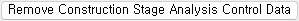Remove the conditions for a construction stage analysis. The construction stage analysis is not performed in this case.

### Frame Output

Calculate Concurrent Forces of Frame : corresponding force components of elements during construction stages. When a max or min value of a particular force component (say strong axis moment) is found, the corresponding other force components (say shear and axial forces) are also calculated.

Calculate Output of Each Part of Composite Section : Check on to calculate stresses and forces pertaining to each of the concrete deck part and the girder part. Otherwise, output will be produced for the total composite section.

### Save Output of Current Stage

The results of the current stage are saved and displayed.

0